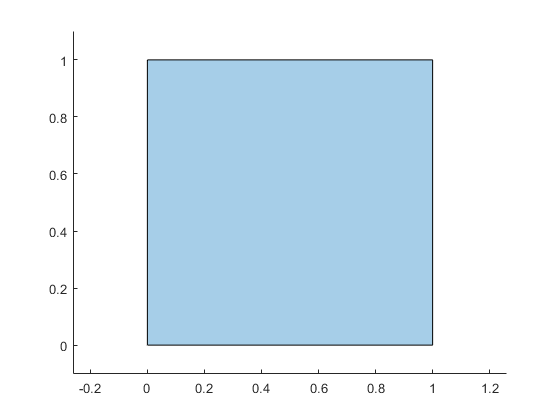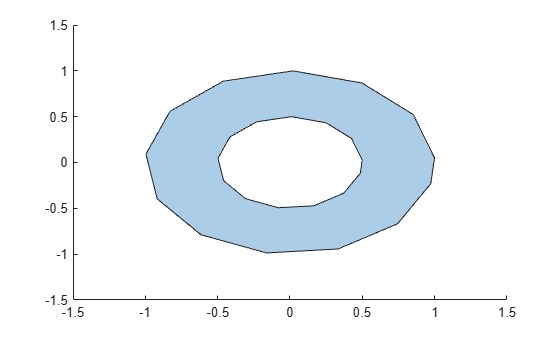# polyshape

## 说明

`polyshape` 函数可创建由二维顶点定义的多边形，并返回具有描述其顶点、实心区域和孔的各种属性的 `polyshape` 对象。例如，`pgon = polyshape([0 0 1 1],[1 0 0 1])` 将创建由四个点 (0,1)、(0,0)、(1,0) 和 (1,1) 定义的实心正方形。

`>> pgon = polyshape([0 0 1 1],[1 0 0 1])`
```pgon = polyshape with properties: Vertices: [4×2 double] NumRegions: 1 NumHoles: 0```
`>> plot(pgon)`## 创建对象

### 语法

``pgon = polyshape()``
``pgon = polyshape(x,y)``
``pgon = polyshape(P)``
``pgon = polyshape(X,Y)``
``pgon = polyshape(___,Name,Value)``

### 描述

``pgon = polyshape()` 创建一个空 `polyshape` 对象。`

``pgon = polyshape(x,y)` 从由 x 坐标向量和对应的 y 坐标向量定义的二维顶点创建 `polyshape`。`x` 和 `y` 的长度必须相同，且至少要有三个元素。`

``pgon = polyshape(P)` 从 N×2 矩阵 `P` 中定义的二维顶点创建 `polyshape`，其中 N 是顶点数。`P` 的第一列定义 x 坐标，第二列定义 `y` 坐标。`

``pgon = polyshape(X,Y)` 中，`X` 和 `Y` 分别是 x 和 `y` 坐标的 1×M 向量元胞数组，该语法创建具有 M 个边界的多边形。`X` 中的每个向量必须与 `Y` 中的对应向量具有相同的长度，但各个边界的顶点数可以互不相同。`

``pgon = polyshape(___,Name,Value)` 为上述任意语法指定创建 `polyshape` 对象的其他参数。`

### 输入参数

`double` 类型的数值输入坐标将自动转换为 `double` 类型。

`double` 类型的数值输入坐标将自动转换为 `double` 类型。

`double` 类型的数值输入坐标将自动转换为 `double` 类型。

M 个边界的 x 坐标，指定为向量元胞数组。每个向量的长度可以不同，但必须与对应的 y 向量的长度匹配。

`double` 类型的数值输入坐标将自动转换为 `double` 类型。

M 个边界的 y 坐标，指定为向量元胞数组。每个向量的长度可以不同，但必须与对应的 y 向量的长度匹配。

`double` 类型的数值输入坐标将自动转换为 `double` 类型。

• `'auto'` - 根据边界嵌套自动确定边界类型。

• `'cw'` - 将顺时针顶点方向视为实心边界。

• `'ccw'` - 将逆时针顶点方向视为实心边界。

• `true` - 当输入顶点产生交集或不正确嵌套时，更改多边形顶点以生成明确定义的多边形。

• `false` - 即使有交集或不正确嵌套，也不更改输入顶点。使用未明确定义的多边形进行计算可能会产生不准确或意想不到的结果。

• `false` - 删除在创建 `polyshape` 时的共线点，使其中包含定义边界所需的最少顶点。

• `true` - 保留在创建 `polyshape` 时的所有共线点作为顶点。

## 对象函数

 `addboundary` 添加 `polyshape` 边界 `polybuffer` 围绕点、线或 `polyshape` 对象创建缓冲区 `rmboundary` 删除 `polyshape` 边界 `rmholes` 删除 `polyshape` 中的孔 `rmslivers` 删除 `polyshape` 边界离群值 `rotate` 旋转 `polyshape` `scale` 缩放 `polyshape` `simplify` 简化 `polyshape` 边界 `sortboundaries` 对 `polyshape` 边界进行排序 `sortregions` 对 `polyshape` 区域进行排序 `translate` 平移 `polyshape`
 `boundary` `polyshape` 边界的顶点坐标 `holes` 将 `polyshape` 孔边界转换为 `polyshape` 对象数组 `ishole` 确定 `polyshape` 边界是否为孔 `isinterior` `polyshape` 内的查询点 `issimplified` 确定 `polyshape` 是否明确定义 `nearestvertex` 查询最近的 `polyshape` 顶点 `numboundaries` `polyshape` 的边界数 `numsides` `polyshape` 的边数 `overlaps` 确定 `polyshape` 对象是否重叠 `plot` 绘制 `polyshape` `regions` 访问 `polyshape` 区域
 `area` `polyshape` 的面积 `boundingbox` `polyshape` 的边界框 `centroid` `polyshape` 的矩心 `convhull` `polyshape` 的凸包 `perimeter` `polyshape` 的周长 `triangulation` 三角剖分 `polyshape` `turningdist` 计算 `polyshape` 对象之间的形变量
 `intersect` `polyshape` 对象的交集 `subtract` 两个 `polyshape` 对象的差集 `union` `polyshape` 对象的并集 `xor` 两个 `polyshape` 对象的异或

## 示例

```pgon = polyshape([0 0 1 3], [0 3 3 0]); plot(pgon)````A = area(pgon)`
```A = 6 ```
`P = perimeter(pgon)`
```P = 10.6056 ```
`[Cx Cy] = centroid(pgon)`
```Cx = 1.0833 ```
```Cy = 1.2500 ```

```P = [0 0; 1 1; 1 0; 0 1; 0 0]; pgon = polyshape(P)```
```Warning: Polyshape has duplicate vertices, intersections, or other inconsistencies that may produce inaccurate or unexpected results. Input data has been modified to create a well-defined polyshape. ```
```pgon = polyshape with properties: Vertices: [7x2 double] NumRegions: 2 NumHoles: 0 ```

`plot(pgon)``A = area(pgon)`
```A = 0.5000 ```

`pgon2 = polyshape(P,'Simplify',false)`
```pgon2 = polyshape with properties: Vertices: [4x2 double] NumRegions: 1 NumHoles: 0 ```
`A2 = area(pgon2)`
```A2 = 0 ```

```t = 0.05:0.5:2*pi; x1 = cos(t); y1 = sin(t); x2 = 0.5*cos(t); y2 = 0.5*sin(t); pgon = polyshape({x1,x2},{y1,y2})```
```pgon = polyshape with properties: Vertices: [27x2 double] NumRegions: 1 NumHoles: 1 ```
`plot(pgon)````x3 = 2*cos(t); y3 = 2*sin(t); pgon2 = addboundary(pgon,x3,y3)```
```pgon2 = polyshape with properties: Vertices: [41x2 double] NumRegions: 2 NumHoles: 1 ```
`plot(pgon2)`